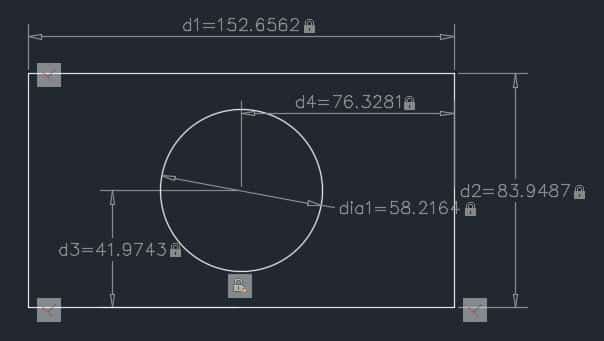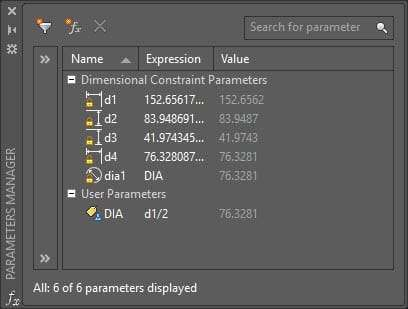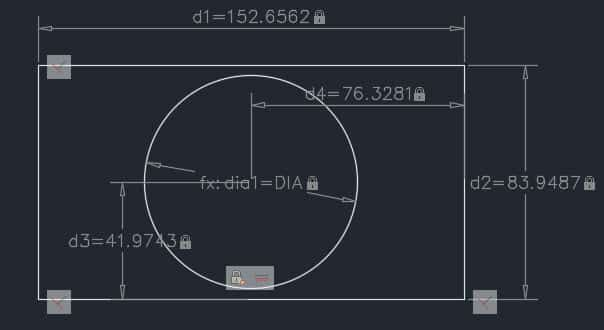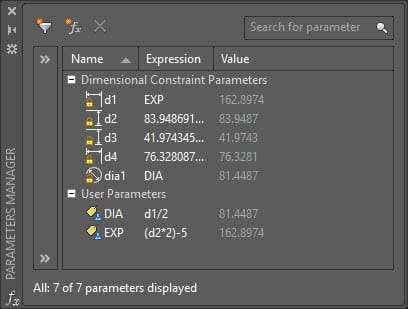The parametric drawing is a method of making geometries with geometrical or dimensional constraints or restrictions. These constraints are applied to the geometry with respect to certain references or other geometries. Parametric drawings update itself if any one parameter is changed, this also helps in maintaining design intent.

This feature of parametric drawing was introduced in 2010 version of AutoCAD. This article explains the concepts of Parametric Drawing, Constraints and Formulas in AutoCAD. Let’s start with applying constraints in the drawing with the help of a simple drawing.

## Applying Constraints:

Make a rectangle with any dimensions and a circle at the geometric center of the rectangle. Now go to the Parametric tab and select the linear option from the Dimensional panel. Click at the top left vertex of the rectangle and then click at the top right vertex and place the linear constraint. Similarly, apply the linear constraint on any one vertical side of the rectangle.Linear constraint on Dimensional panel

The dimensional constraint is different from the simple dimensioning and should not be confused with that. Select Diameter constraint from Dimensional panel and apply it to the circle at the geometric center of the rectangle. Now apply the linear constraint to the circle with respect to the horizontal and verticle side of the rectangle.

Now select Fix constraint from the Geometric panel and click at two diagonally opposite vertices of the rectangle to make it fixed. Now select Perpendicular constraint and apply it on three vertices of the rectangle. The geometry is now fully constrained as shown in the image below.## Making simple formulas:

Let’s assume that we want the geometry made above to behave in such a way that diameter of the circle always remains half of length of the rectangle. For that, we can create a simple relation or formula and apply it to the respective geometries in the drawing.

Click on Parameters Manager icon on Manage panel of Parameters tab or use its command equivalent PARAMETERS. Click on theicon on Parameters Manager palette, a new list User Parameters will be added to the palette.Parameters manager palette

Double click on the user1 parameter and change its name to DIA. Double click again on expression column next to DIA and type d1/2 which mean DIA parameter will be equal to d1/2 where d1 is the length of the Rectangle.

You can now use this formula to define the diameter of the circle, double click on Expression column of dia1 row in parameters manager palette and change it to DIA as shown in the image above, the circle will update according to changed dimension and diameter of circle will become equal to value of DIA which is half of length of rectangle.To test this formula go to d1 in drawing area and change its value, you will notice that diameter of the circle will also change accordingly not only in parameters manager palette but also in the drawing area.

## Making nested formulas:

In the above example, I have used a simple formula but you can also use nested and complex formulas that involve the use of sine, cosine and logarithmic expressions. I will use the same example of rectangle and circle to explain this as well.

Suppose we want to change the length of the rectangle (d1) in such a way that it always remains five units less than twice of the width of the Rectangle (d2) then we can use this expression (d2*2)-5 to define the length of the rectangle where d2 is the width of the Rectangle.

To apply this formula click on theicon and double click on user2 and rename it to EXP. Again double click on the expression box next to EXP and write the expression (d2*2)-5.

Now write EXP in d1 row expression column as shown in the image below. You will notice that length of rectangle changes to reflect dimension according to formula. Final settings in parameters manager will look like this.The final parameters in Parameters manager palette

You can test this nested expression by changing the value of d2 which will change dimensions of all dependent geometries including the diameter of the circle.

Do you have questions related to this tip? Let me know in comments below.